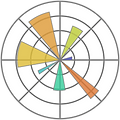# Data Science Essentials

###### Level: Beginner###### Syllabus

1. Introduction to data science

• What is data science

• What are companies looking for

• How it helps businesses to make right the decisions

• What tools are used

• Installing Python and Jupyter with Anaconda

2. Python fundamentals - I

• Importing libraries

• Variables and data types

• Lists

• Dictionary

3. Python Fundamentals - II

• Conditional statements

• Functions

• Loops

4. Pandas and Numpy fundamentals

• Why pandas and numpy

• Importing Pandas and Numpy

• Exploring data with pandas

• Boolean indexing

5. Statistics and Probability

• Descriptive statistics

• Probability concepts

• Random variables

• Probability distribution functions

• Central Limit Theorem (CLT)

6. Linear Algebra

• Linear combinations

• Vectors and Matrices

• Matrix Decomposition

• Eigen Vectors and Eigen Values

7. Data Cleaning and Analysis

• Missing values

• Duplicate data

• Working with strings

• Grouping and combining

8. Data visualization in Python - I

• Matplotlib basics

• Line charts

• Bar plots

• Scatter Plots

• Histogram and box plots

9. Data visualization in Python - II

• Seaborn Basics

• Line plots

• Bar plots

• Scatter plots

• Histogram and box plots

10. Project

• Problem Statement

• Exploratory Data Analysis

• Data Cleaning

• Data Visualization

• Insights and Conclusion

# \$1599